NameFlagsCard.TypeDescription & Constraints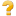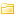Extension 0..1ExtensionExtension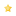extension 0..0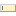url 1..1uri"http://fhir.ch/ig/ch-core/StructureDefinition/ch-ext-ech-10-linetype"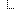valueCode 0..1codeValue of extension
Binding: eCH-010 Address Line Type (required): A set of codes advising a system or user which name in a set of names to select for a given purpose.Documentation for this format
<status value="active"/> <date value="2018-11-27"/> <publisher value="HL7 Switzerland"/> <contact> <name value="HL7 Switzerland"/> <telecom> <system value="url"/> <value value="https://www.hl7.ch/"/> </telecom> </contact> <description value="eCH-0010: Extension to define line types for addresses"/> <jurisdiction> <coding> <system value="urn:iso:std:iso:3166"/> <code value="CH"/> </coding> </jurisdiction> <copyright value="CC-BY-SA-4.0"/> <fhirVersion value="4.0.1"/> <mapping> <identity value="eCH"/> <uri value="https://www.ech.ch/"/> <name value="eCH-0010 Datenstandard Postadresse"/> </mapping> <mapping> <identity value="rim"/> <uri value="http://hl7.org/v3"/> <name value="RIM Mapping"/> </mapping> <kind value="complex-type"/> <abstract value="false"/> <context> <type value="element"/> <expression value="Address.line"/> </context> <type value="Extension"/> <baseDefinition value="http://hl7.org/fhir/StructureDefinition/Extension"/> <derivation value="constraint"/> <snapshot> <element id="Extension"> <path value="Extension"/> <short value="Extension"/> <definition value="eCH-0010: Extension to define line types for an address"/> <min value="0"/> <max value="1"/> <base> <path value="Extension"/> <min value="0"/> <max value="*"/> </base> <condition value="ele-1"/> <constraint> <key value="ele-1"/> <severity value="error"/> <human value="All FHIR elements must have a @value or children"/> <expression value="hasValue() or (children().count() > id.count())"/> <xpath value="@value|f:*|h:div"/> <source value="http://hl7.org/fhir/StructureDefinition/Element"/> </constraint> <constraint> <key value="ext-1"/> <severity value="error"/> <human value="Must have either extensions or value[x], not both"/> <expression value="extension.exists() != value.exists()"/> <xpath value="exists(f:extension)!=exists(f:*[starts-with(local-name(.), 'value')])"/> <source value="http://hl7.org/fhir/StructureDefinition/Extension"/> </constraint> <isModifier value="false"/> </element> <element id="Extension.id"> <path value="Extension.id"/> <representation value="xmlAttr"/> <short value="Unique id for inter-element referencing"/> <definition value="Unique id for the element within a resource (for internal references). This may be any string value that does not contain spaces."/> <min value="0"/> <max value="1"/> <base> <path value="Element.id"/> <min value="0"/> <max value="1"/> </base> <type> <extension url="http://hl7.org/fhir/StructureDefinition/structuredefinition-fhir-type"> <valueUrl value="string"/> </extension> <code value="http://hl7.org/fhirpath/System.String"/> </type> <isModifier value="false"/> <isSummary value="false"/> <mapping> <identity value="rim"/> <map value="n/a"/> </mapping> </element> <element id="Extension.extension"> <path value="Extension.extension"/> <slicing> <discriminator> <type value="value"/> <path value="url"/> </discriminator> <description value="Extensions are always sliced by (at least) url"/> <rules value="open"/> </slicing> <short value="Extension"/> <definition value="An Extension"/> <min value="0"/> <max value="0"/> <base> <path value="Element.extension"/> <min value="0"/> <max value="*"/> </base> <type> <code value="Extension"/> </type> <constraint> <key value="ele-1"/> <severity value="error"/> <human value="All FHIR elements must have a @value or children"/> <expression value="hasValue() or (children().count() > id.count())"/> <xpath value="@value|f:*|h:div"/> <source value="http://hl7.org/fhir/StructureDefinition/Element"/> </constraint> <constraint> <key value="ext-1"/> <severity value="error"/> <human value="Must have either extensions or value[x], not both"/> <expression value="extension.exists() != value.exists()"/> <xpath value="exists(f:extension)!=exists(f:*[starts-with(local-name(.), "value")])"/> <source value="http://hl7.org/fhir/StructureDefinition/Extension"/> </constraint> <isModifier value="false"/> <isSummary value="false"/> </element> <element id="Extension.url"> <path value="Extension.url"/> <representation value="xmlAttr"/> <short value="identifies the meaning of the extension"/> <definition value="Source of the definition for the extension code - a logical name or a URL."/> <comment value="The definition may point directly to a computable or human-readable definition of the extensibility codes, or it may be a logical URI as declared in some other specification. The definition SHALL be a URI for the Structure Definition defining the extension."/> <min value="1"/> <max value="1"/> <base> <path value="Extension.url"/> <min value="1"/> <max value="1"/> </base> <type> <code value="uri"/> </type> <fixedUri value="http://fhir.ch/ig/ch-core/StructureDefinition/ch-ext-ech-10-linetype"/> <isModifier value="false"/> <isSummary value="false"/> <mapping> <identity value="rim"/> <map value="N/A"/> </mapping> </element> <element id="Extension.value[x]"> <path value="Extension.value[x]"/> <slicing> <discriminator> <type value="type"/> <path value="\$this"/> </discriminator> <ordered value="false"/> <rules value="closed"/> </slicing> <short value="Value of extension"/> <definition value="Value of extension - must be one of a constrained set of the data types (see [Extensibility](http://hl7.org/fhir/R4/extensibility.html) for a list)."/> <min value="0"/> <max value="1"/> <base> <path value="Extension.value[x]"/> <min value="0"/> <max value="1"/> </base> <type> <code value="code"/> </type> <constraint> <key value="ele-1"/> <severity value="error"/> <human value="All FHIR elements must have a @value or children"/> <expression value="hasValue() or (children().count() > id.count())"/> <xpath value="@value|f:*|h:div"/> <source value="http://hl7.org/fhir/StructureDefinition/Element"/> </constraint> <isModifier value="false"/> <isSummary value="false"/> <mapping> <identity value="rim"/> <map value="N/A"/> </mapping> </element> <element id="Extension.value[x]:valueCode"> <path value="Extension.value[x]"/> <sliceName value="valueCode"/> <short value="Value of extension"/> <definition value="Value of extension - may be a resource or one of a constrained set of the data types (see Extensibility in the spec for list)."/> <min value="0"/> <max value="1"/> <base> <path value="Extension.value[x]"/> <min value="0"/> <max value="1"/> </base> <type> <code value="code"/> </type> <constraint> <key value="ele-1"/> <severity value="error"/> <human value="All FHIR elements must have a @value or children"/> <expression value="hasValue() or (children().count() > id.count())"/> <xpath value="@value|f:*|h:div"/> <source value="http://hl7.org/fhir/StructureDefinition/Element"/> </constraint> <isModifier value="false"/> <isSummary value="false"/> <binding> <strength value="required"/> <description value="A set of codes advising a system or user which name in a set of names to select for a given purpose."/> <valueSet value="http://fhir.ch/ig/ch-core/ValueSet/ech-10-linetype"/> </binding> <mapping> <identity value="rim"/> <map value="N/A"/> </mapping> </element> </snapshot> <differential> <element id="Extension"> <path value="Extension"/> <short value="Extension"/> <definition value="eCH-0010: Extension to define line types for an address"/> <min value="0"/> <max value="1"/> </element> <element id="Extension.extension"> <path value="Extension.extension"/> <max value="0"/> </element> <element id="Extension.url"> <path value="Extension.url"/> <type> <code value="uri"/> </type> <fixedUri value="http://fhir.ch/ig/ch-core/StructureDefinition/ch-ext-ech-10-linetype"/> </element> <element id="Extension.valueCode"> <path value="Extension.valueCode"/> <short value="Value of extension"/> <definition value="Value of extension - may be a resource or one of a constrained set of the data types (see Extensibility in the spec for list)."/> <min value="0"/> <max value="1"/> <base> <path value="Extension.value[x]"/> <min value="0"/> <max value="1"/> </base> <type> <code value="code"/> </type> <isModifier value="false"/> <isSummary value="false"/> <binding> <strength value="required"/> <description value="A set of codes advising a system or user which name in a set of names to select for a given purpose."/> <valueSet value="http://fhir.ch/ig/ch-core/ValueSet/ech-10-linetype"/> </binding> </element> </differential> </StructureDefinition>